# Team:SCUT/Modeling/Odr-10 Pathway# ODE pathway analysis

Aim:
To model the feasibility of the odr-10 pathway.
Step:

1. Build up the equations between variable quantity based on Mass-action law
2. Investigate reasonable parameter sets from previous learning and researches
3. Run the model and simulation
4. Verify the feasibility of the pathway model

Background:
The yeast pheromone pathway is one of the best understood and described pathways in eukaryotes. This pheromone signaling system can be divided into three modules: G-protein cycle activation, the MAPK cascade and the downstream effects of activated Fus3.
In the pheromone pathway, the input (extra inducer concentration) controls [P], we formulate the mechanism above quantitatively via kinetic model (ODE model) for the pathway. The model is cast in terms of mass-action equations for the cellular concentrations.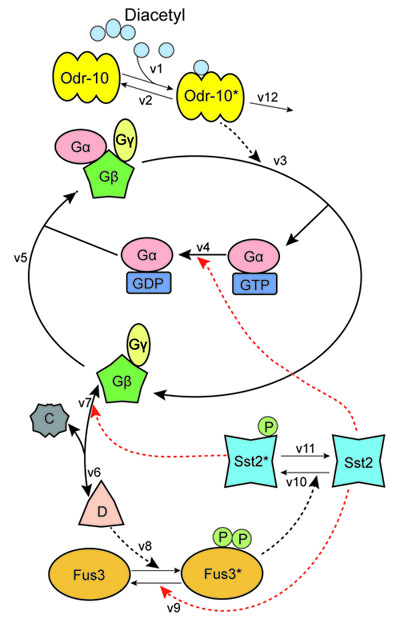Figure 1. Odr-10 pathway

"[ ]" stands for concentration;
"k" stands for the reaction constant

ODE pathway..

Parameter set..

Parameter sensitivity..

Parameter sweep

Reference

Parameter set:
In the ODE systems, the parameters are uncertain. We verified the feasibility of constructing such a device through ODE simulation, using the parameter set from previous researches.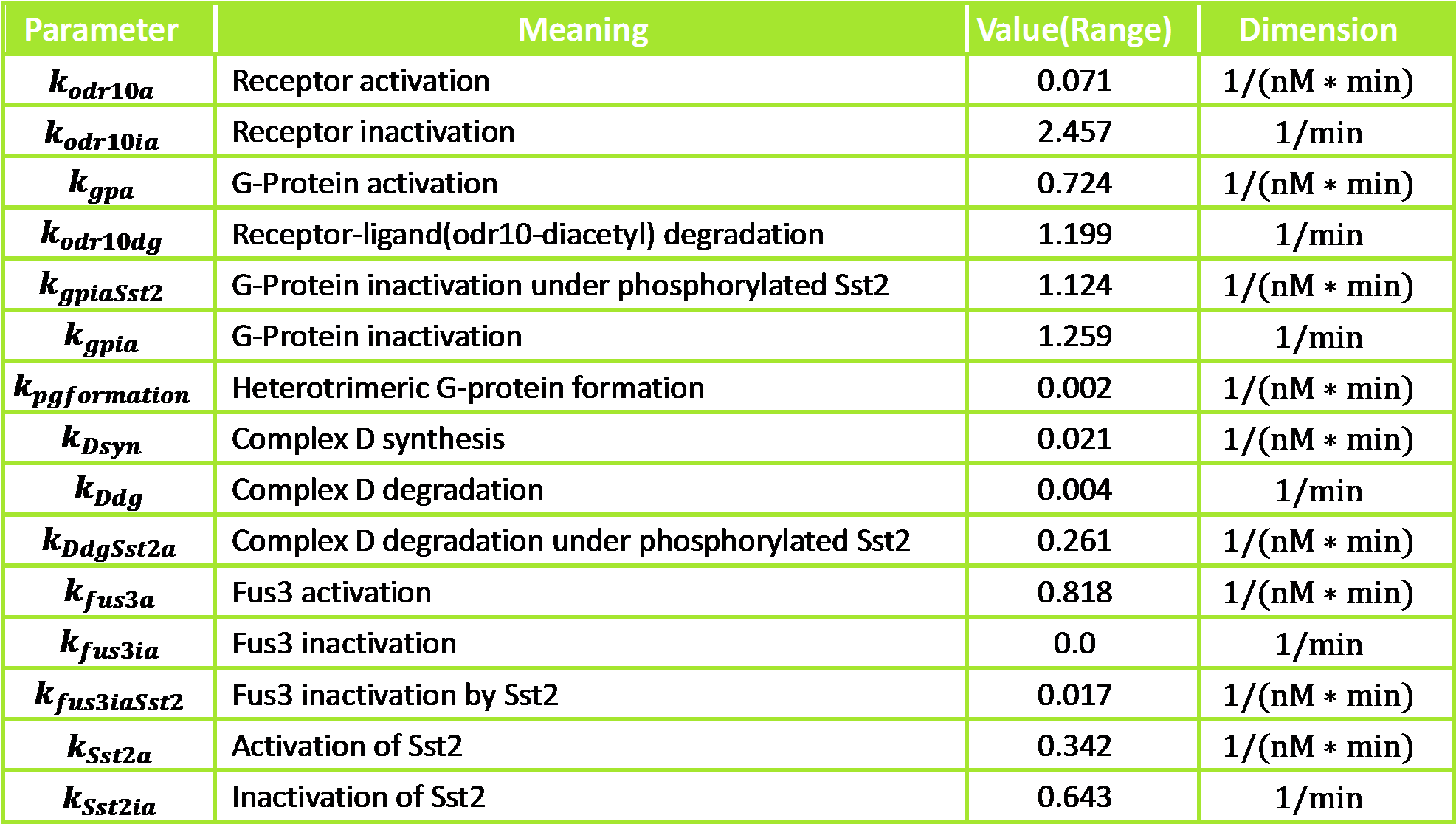Figure 2. Parameter set of odr-10 pathway ODE simulation

The simulation result:

Comparator (knockout the Sst2 gene) odr-10 pathway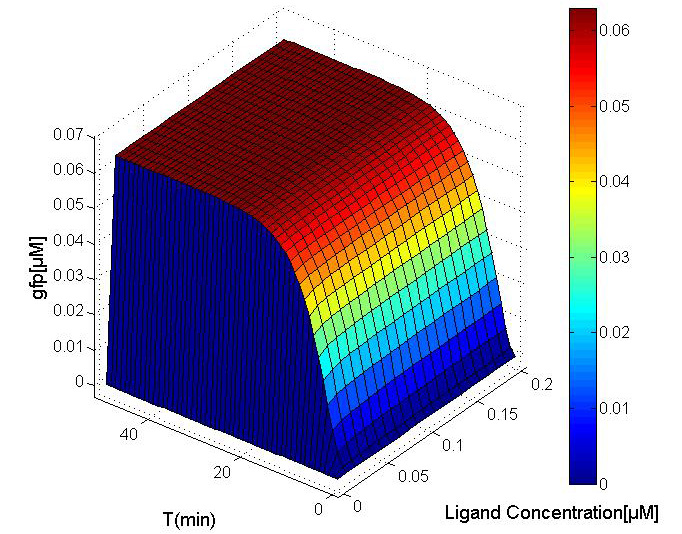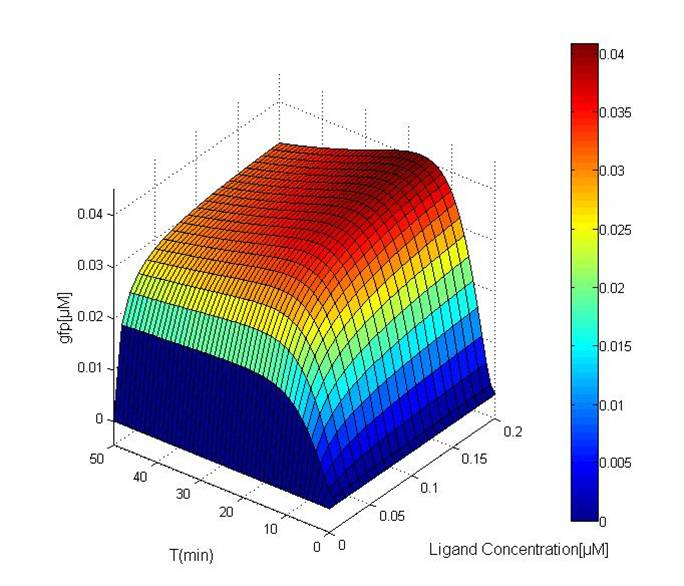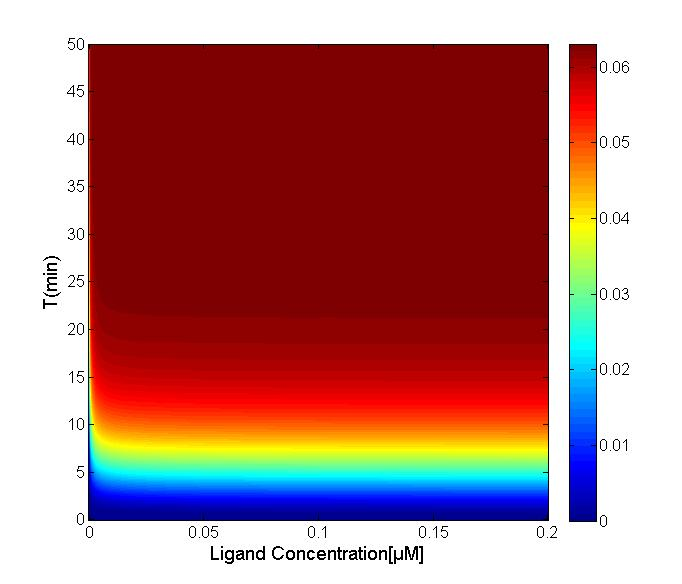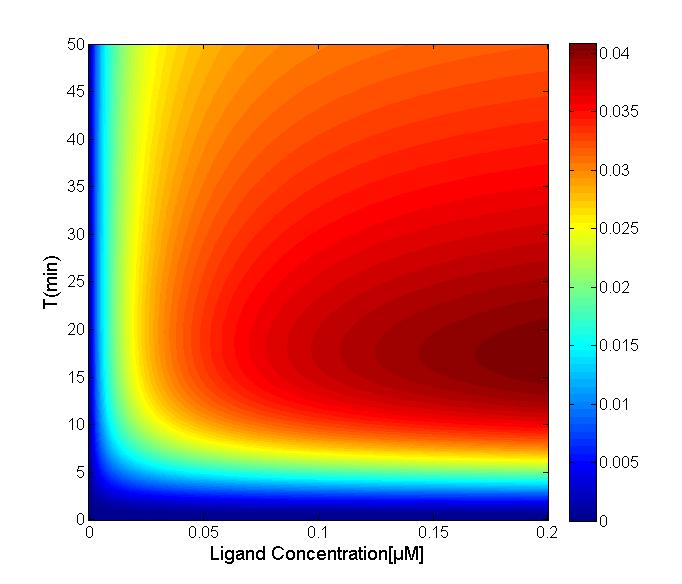For the Comparator, simple conclusions could be drawn:

1. When [diacetyl] = 0, mature[GFP] = 0;
2. When [diacetyl] 0, mature[GFP] = 0.06.

According to the simulation result, the pathway, which knockout Sst2 gene, is sensitive. And it isn't suitable for being the receptor of oscillation producer.
It is worthy to emphasize that a major negative regulator of the pathway, Sst2, activating factors that return GαGTP to its inactive state, GαGDP, which generally would be deleted to increase the sensitivity. However, depend modeling analysis, we find that Sst2 gene knockout would result in pathway to be extremely sensitivity which is beyond our expectation.
Discussing the ODE pathway model are established based on Adriana Supady. We have made a further development. In addition, our model has considered the influence of Ste12 and the transcription and translation rate of G-protein. On the other hand, we figure out the change of model without the Sst2, and aid to the experiment of odr-10 pathway. Anyway, to a large degree, we can't complete the model without the achievement of Adriana Supady and others.

# Parameter sensitivity analysis

Aim: To figure out the sensitive value of parameters in the model.
Steps:

1. Parameter range determination;
2. Analyze the sensitivity of parameters with local sensitivity method;
3. Determine important parameters for parameter sweep.

We need to figure out which parameter is playing a significant role in the odr-10 pathway model, and we will try to further our optimization of parameter set found in ODE equations.
Local sensitivity analysis is a common approach that the sensitivity of a model output.
The systems biology models is a system of ODE that is dependent on a certain parameter set p and initial conditions yi(0).The sensitivity coefficients are the first-order derivatives of model outputs with respect to model parameters:Where yi is the ith model output and p is the model input parameter.Why should we choose slope as an indicator?
t = constantAt the same time, slope is not the only candidate indicator; the other indicator candidates are listed below:Result:
Parameters sensitivity for odr-10 pathway model: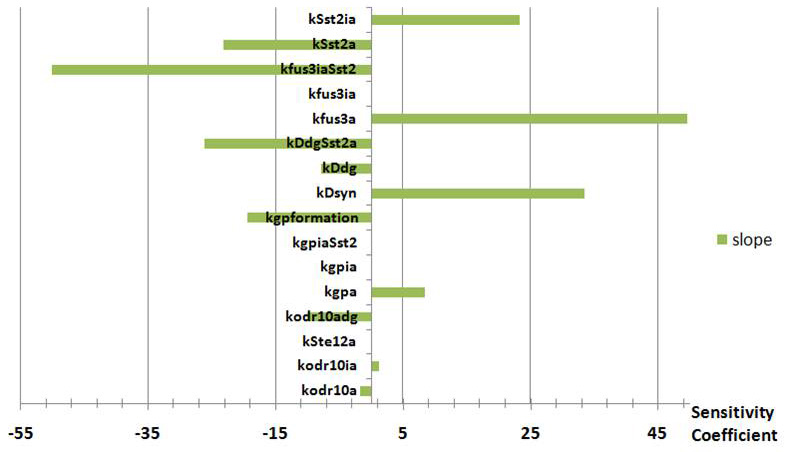Figure 3. The histogram of parameter sensitivity for odr-10 pathway

Positive (negative) values of calculated sensitivities indicate that the final concentration of Fus3* increase (decrease) with the increase of the parameter value.
The highest absolute value has been calculated for parameter kfus3iaSst2 that represents the positive feedback of Sst2 acting on the deactivation of Fus3*. Another parameter that has a great impact on the final concentration of the output ([GFP]) is kfus3a that represents the activation of Fus3.

# Parameter sweep

Aim: To discover the optimized value of important parameters and the best result of our model.
Steps:

1. Choose indicator
2. Determine important parameters for sweeping by sensitivity analysis
3. Simulation and establish databases
4. Draw the heat maps of project
5. Determine optimal parameter value

Result:
We determine the optimal parameter ranges for the ODE pathway system, and select a best parameter set for the noise analysis.
We sweep sensitive parameters for the follow reasons: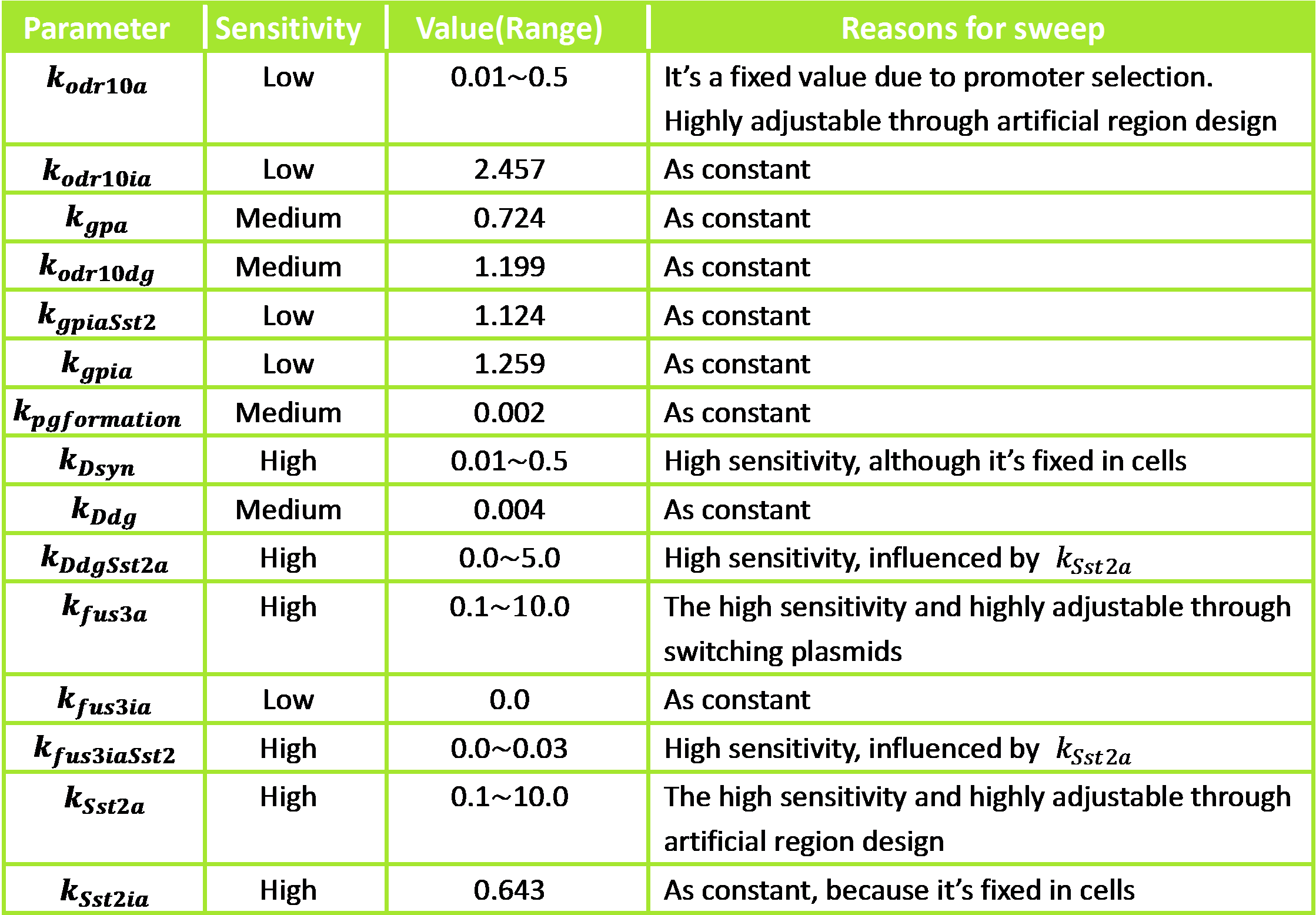Establish parameter sweep database
For the database is a collection of 5-Dimensional data points, it's easy to show the result in the format of projection on 2-Dimensional surface to indicate the relationship between [GFP] and arbitrary 2 parameters we sweep.

Pathway of odr-10 ( [GFP] vs arbitrary 2 parameters )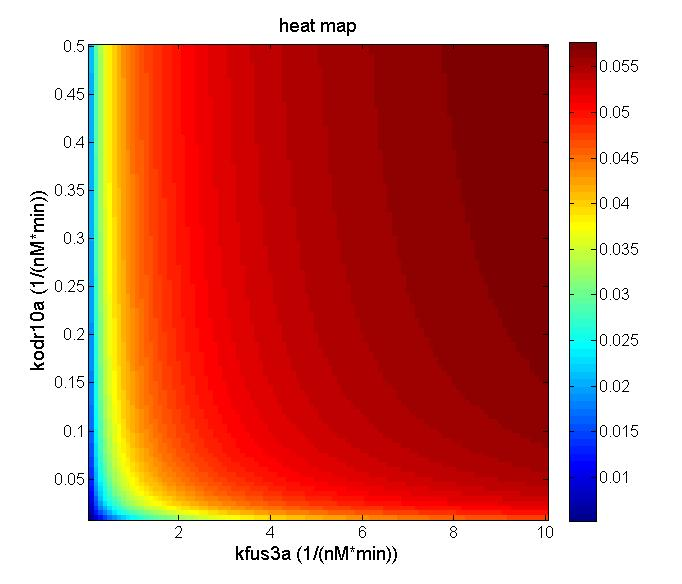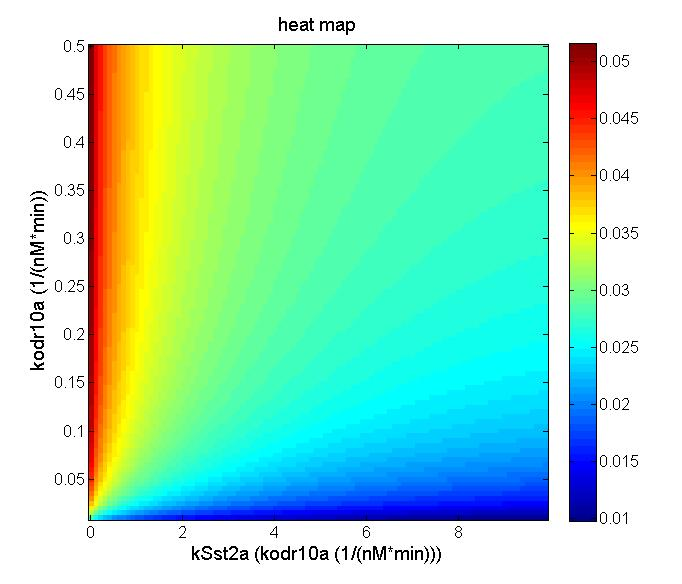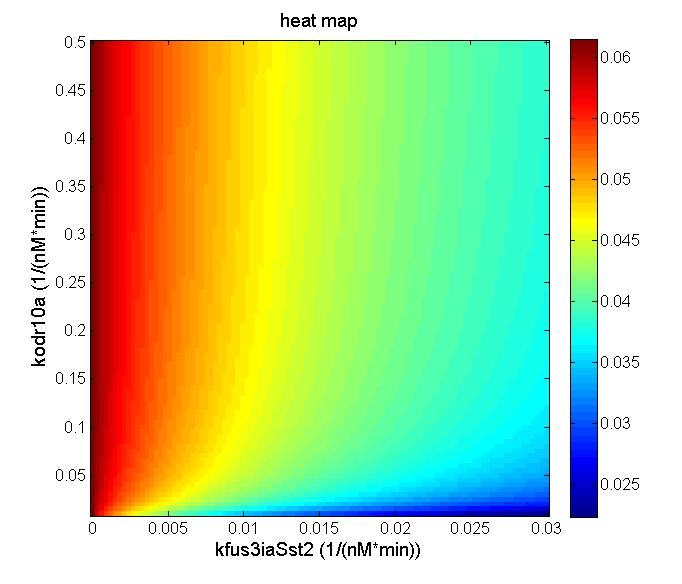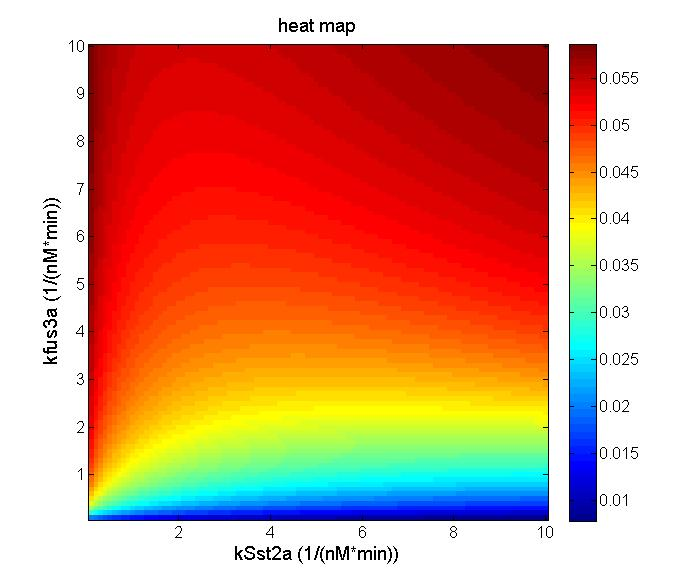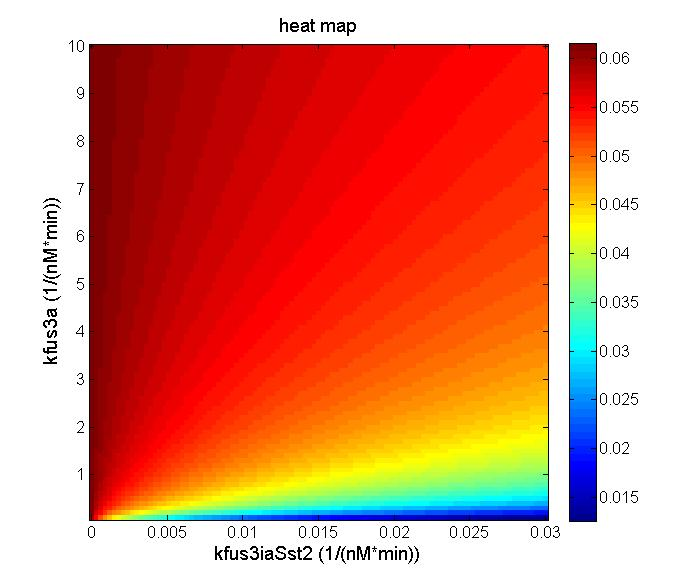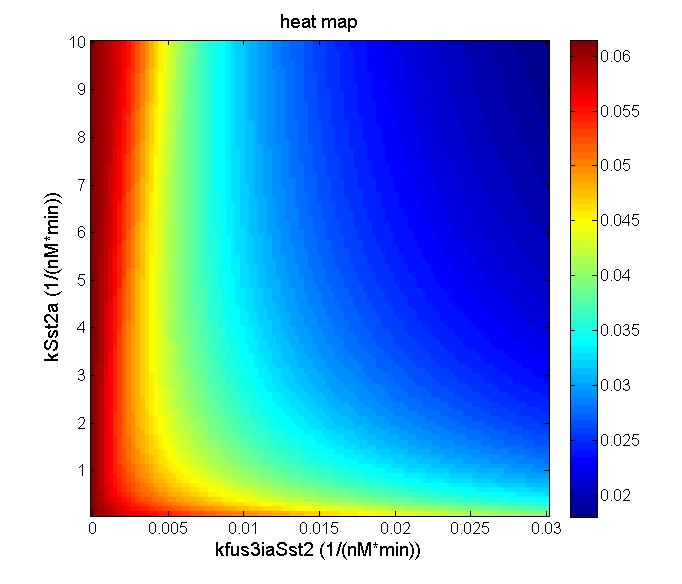Determine optimal parameter range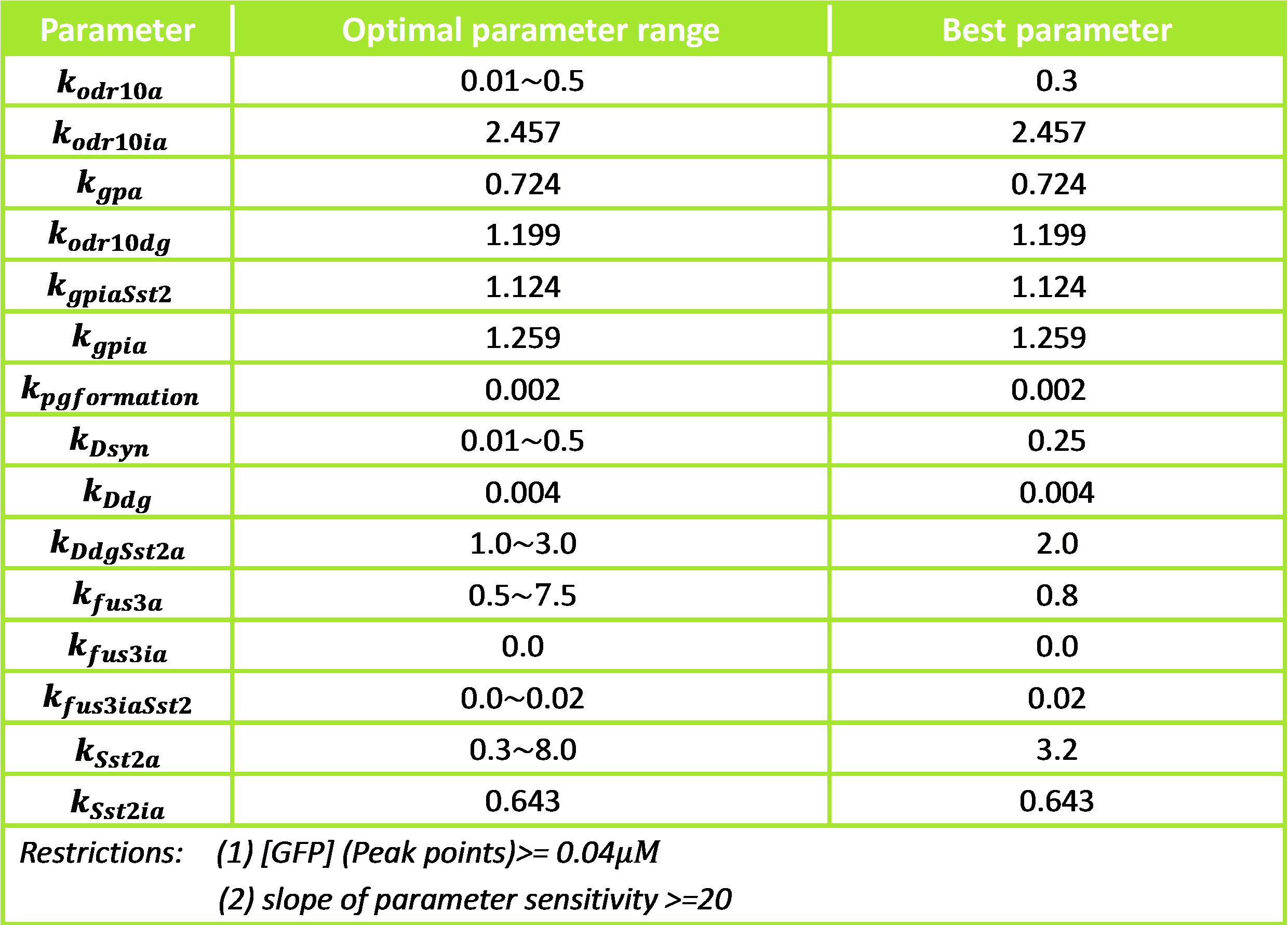We will use the best parameter set to perform the noise analysis.

Noise analysis-model:
Aim: To find out whether the ODE pathway system is steady.
Steps:

1. Using the Gillespie algorithm to analysis the stochastic model.
2. Drawing the probability distribution figure is to observe the result whether it is around the certainly value.
3. Simulation and computing the noise result
4. Comparing the noise result

Model preparation:
In the biological reactions, the noise plays an important role in affecting the system and it is meaningful for us to have noise analysis. And we refer to the most classical Gillespie algorithm.
On the other hand, lots of improvements have been made by later generation. For example, the Dynamic Monte Carlo methods are a common means of simulating the time-evolution of chemical systems.
The Gillespie Algorithm (SSA) is the standard algorithm for noise analysis.
We use Gillespie algorithm for some reasons:

1. The stochastic simulation algorithm is exact.
2. The stochastic simulation program will normally require little in the way of computer memory space.
3. The stochastic simulation algorithm essentially provides us with information on the time of individual ensemble members, such as one would observe in an ideal laboratory experiment.

The construction of model:
Through the parameter data of parameter sweep, we get the following graphics by using Gillespie algorithm. ψij is use to describe the noise.σij stands for standard deviation and < xi > represents the mean value
if i=j,We can figure out the value of ψi2 by getting the answers of its mean value and variance.

Result:
To get how the odr-10 system behaves in the stochastic model, we plot the mRNA and G-protein molecule as function of time, and we draw the histogram. The histogram makes us clear that the stochastic value is around the mean, so we can conclude that our odr-10 system is stable.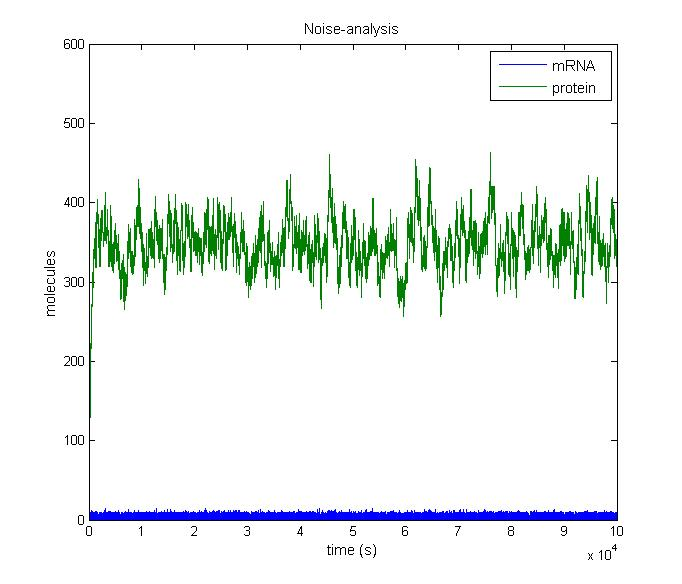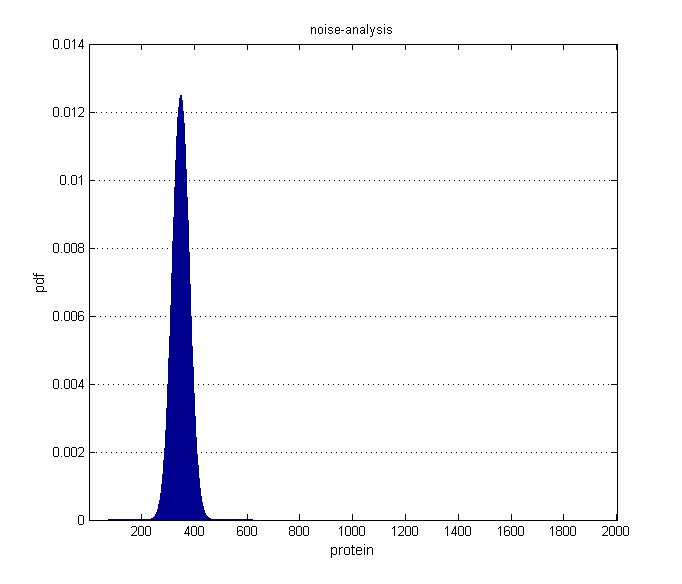Figure 4. The parameters which are from the parameter sweep are used in the Gillespie algorithm. The left is the mRNA and protein change with time goes by. The right is the protein of probability distribution frequency (pdf).

Conclusion:
Through the noise analysis, we can conclude that the protein isn't drawn the noise. And our model also meets the requirements to design the experiment.

# Reference:

 Tau-Mu Yi, Hiroaki Kitano, and Melvin I. Simon, A quantitative characterization of the yeast heterotrimeric G protein cycle, PNAS September 16, 2003 Vol. 100 No.19.
 Kofahl B, Klipp E, Modelling the dynamics of the yeast pheromone pathway, Yeast 2004; 21: 831-850.
 Adriana Supady, Theoretical and computational analysis of negative feedback mechanisms and the dose-response alignment in the pheromone signalling pathway of the yeast Saccharomyces cerevisiae.
 Z.Zi, Sensitivity analysis approaches applied to systems biology models, IET Syst. Biol, 2011, Vol. 5, Iss. 6, pp. 336-346.
 Ozbudak, E. M., Thattai, M., Kurtser, I., Grossman, A. D. & van Oudenaarden, Regulation of noise in the expression of a single gene, Nature Genet. 31, 69-73 (2002).